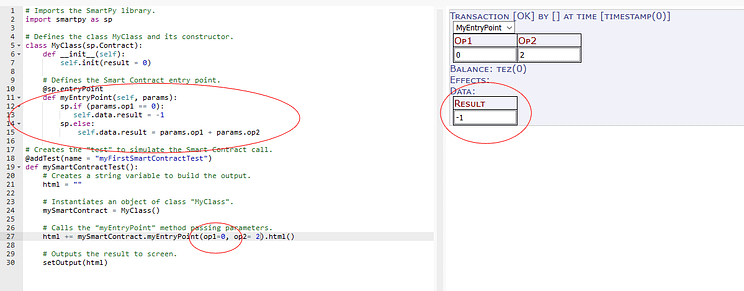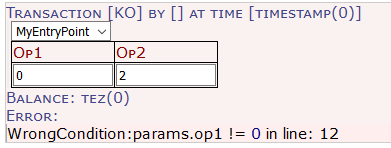# How to write Smart Contracts for Blockchain using Python (Part 3)Share :```# Imports the SmartPy library.
import smartpy as sp# Defines the class MyClass and its constructor.
class MyClass(sp.Contract):
def __init__(self):
self.init(result = 0)

# Defines the Smart Contract entry point.
@sp.entryPoint
def myEntryPoint(self, params):
self.data.result = params.op1 + params.op2

# Creates the "test" to simulate the Smart Contract call.
def mySmartContractTest():
# Creates a string variable to build the output.
html = ""

# Instantiates an object of class "MyClass".
mySmartContract = MyClass()

# Calls the "myEntryPoint" method passing parameters.
html += mySmartContract.myEntryPoint(op1 = 1, op2 = 2).html()

# Outputs the result to screen.
setOutput(html)```
```    sp.if (params.op1 == 0):
self.data.result = -1
sp.else:
self.data.result = params.op1 + params.op2```Result from running the script with an “if-else” statement
```sp.verify(params.op1 != 0)
self.data.result = params.op1 + params.op2```Using condition checking with the “verify” statement
```sp.for i in sp.range(0, 5):
self.data.result += params.op1 + params.op2```
```@sp.entryPoint